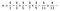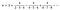# Using Python To Play With Pi(π)

## Analyzing the efficiency of the two most well-known Pi series with Matplotlib and PIL

Hello! It’s a pleasure to have you here. Here’s what I’ll cover:

• Presentation and compaction of two most well-known Pi series.
• Construction of an algorithm to measure the efficiency of these Pi series.
• Matplotlib for plot graphs of returned values from the algorithm.
• PIL for grouping the graphics into a gif.

Observation: This is NOT a tutorial.

Pi(π). Probably, when someone talks about it, a lot of formulas that you learned in high school come up to your mind as the circumference length or maybe the sphere volume. But here, I won’t be talking about it. My intention is to show the beauty and potential of code lines.

Just a little presentation: Pi is an irrational number with infinite decimal places considered by some mathematicians the most important number in the universe. This elegant and mysterious number just made the GPS possible and it’s present in almost all NASA operations.

I don’t intend to stay in Pi utilities. I want to focus on the different ways of defining it. Following are the most known ones:

What I’m going to be doing here is contemplating and exploring those two series using the power that computers provide us. My goal is to build an algorithm that calculates and records the stopping point(position of the last added term of the series) along with the number of correct decimal places of pi.

# MATH PART

Here’s what will be covered in this part:

Serie → Summation Expression → Algorithm

First Step: Pi Serie → Summation Expression

I could take Leibniz Series and Nilakantha Series to start soon building my algorithm. But, why don’t use math to shorten the way? It can be done just by compacting the formula in a summation expression. Like this:

Compacting Leibniz Series:Leibniz Series

Compacting Nilakantha Series:Nilakantha Series

The typical elements will be the most essential part of our algorithm.

Second Step: Summation Expression → Algorithm

We’re not in the code yet. Let’s use a few fundamentals and logic of programming to turn the summation expression terms into loop structure terms. Here is it:

That’s enough! Let’s code!

# THE ALGORITHM CONSTRUCTION WITH PYTHON

First of all, we need to import the math and decimal libraries, both are native from Python. Math will be used to get the Pi value and Decimal to deal with the high-precision of decimal places.

To lighten a little, let’s build a method for the Nilakila Series that calculates up to the stopping point number one hundred and fifty thousand and records the accuracy of decimals places. When a new correct decimal place is “found”, the method will print the stopping point and the accuracy.

The output:

Now, instead of printing, let’s reform the function so that it stores the values ​​in two lists (stopping point list and decimal places accuracy list) for each series.

Applying the reformed function to the two series, we will have a total of four lists witch are: one stopping points list for Nilakila, one stopping points list for Leibniz, one decimal accuracy list for Nilakila, and one decimal accuracy list for Leibniz.

# VISUALIZATION WITH MATPLOTLIB AND PILLOW

Now, what we have to do is equalize the number of terms in the lists to provide better visualization in the graphs. After, plot 26 graphs with matplotlib, and grouping those graphs in a gif with PIL.